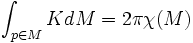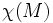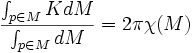# Gauss-Bonnet theorem for surfaces

This result relates information on curvature to information on topology of a manifold

This result is about manifolds in dimension:2

## Statement

The Gauss-Bonnet theorem states that the average value of Gaussian curvature over a volume-normalized compact orientable two-dimensional Riemannian manifold is proportional to the Euler characteristic of the manifold. Specifically, if$K$ denotes the Gaussian curvature at point$p \in M$,then:$\int_{p \in M} K dM = 2\pi\chi(M)$

Here$\chi(M)$ denotes the Euler characteristic, which is a purely topological notion.

Note that the Gauss-Bonnet theorem works only for orientable manifolds since it crucially depends on an embedding in 3-space.

When the manifold is not volume-normalized, we have the formula:$\frac{\int_{p \in M} K dM}{\int_{p \in M} dM} = 2\pi \chi(M)$

## Importance

### As a tool for area computation

The Gauss-Bonnet theorem can be used to compute areas of regions in surfaces having constant curvature. For instance, it can be used to obtain a formula for the area of a geodesic triangle on the two-dimensional sphere, embedded in three-space.

### As a topological control on the Gaussian curvature

The Gauss-Bonnet theorem tells us that given a manifold, we cannot give it both a metric with everywhere positive Gaussian curvature, and a metric with everywhere negative curvature.

It also tells us that if we fix the total volume of the manifold, then there is only one possible value for the curvature, if it is constant.

## Related facts

### Gauss-Bonnet theorem for hypersurfaces

Further information: Gauss-Bonnet theorem for hypersurfaces

This is a generalization of the Gauss-Bonnet theorem to hypersurfaces. The Gaussian curvature is replaced by the Gauss-Kronecker curvature.

### Riemann-Roch theorem

Further information: Riemann-Roch theorem

The Gauss-Bonnet theorem, which is a tool for computation of the Euler characteristic, can be viewed as a special case of the Hirzebruch-Riemann-Roch theorem, which is a more general version of the Riemann-Roch theorem for curves. Let's see what this means:

• A result of Riemann-Roch type is one that computes an Euler characteristic-type quantity (which is a sum (or difference) of dimensions of certain vector spaces), in terms of certain characteristic classes
• The Gauss-Bonnet theorem computes the Euler characteristic as an integral of a curvature over a region. It is of Riemann-Roch type because the integral of curvature can be viewed as arising from certain characteristic classes.